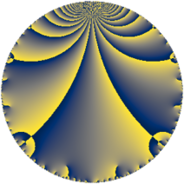# Properties

 Label 230.4.aLevel $230$ Weight $4$ Character orbit 230.a Rep. character $\chi_{230}(1,\cdot)$ Character field $\Q$ Dimension $22$ Newform subspaces $10$ Sturm bound $144$ Trace bound $3$

# Related objects

## Defining parameters

 Level: $$N$$ $$=$$ $$230 = 2 \cdot 5 \cdot 23$$ Weight: $$k$$ $$=$$ $$4$$ Character orbit: $$[\chi]$$ $$=$$ 230.a (trivial) Character field: $$\Q$$ Newform subspaces: $$10$$ Sturm bound: $$144$$ Trace bound: $$3$$ Distinguishing $$T_p$$: $$3$$

## Dimensions

The following table gives the dimensions of various subspaces of $$M_{4}(\Gamma_0(230))$$.

Total New Old
Modular forms 112 22 90
Cusp forms 104 22 82
Eisenstein series 8 0 8

The following table gives the dimensions of the cuspidal new subspaces with specified eigenvalues for the Atkin-Lehner operators and the Fricke involution.

$$2$$$$5$$$$23$$FrickeDim.
$$+$$$$+$$$$+$$$$+$$$$4$$
$$+$$$$+$$$$-$$$$-$$$$2$$
$$+$$$$-$$$$+$$$$-$$$$2$$
$$+$$$$-$$$$-$$$$+$$$$4$$
$$-$$$$+$$$$+$$$$-$$$$1$$
$$-$$$$+$$$$-$$$$+$$$$4$$
$$-$$$$-$$$$+$$$$+$$$$4$$
$$-$$$$-$$$$-$$$$-$$$$1$$
Plus space$$+$$$$16$$
Minus space$$-$$$$6$$

## Trace form

 $$22 q - 4 q^{2} + 16 q^{3} + 88 q^{4} + 40 q^{6} + 8 q^{7} - 16 q^{8} + 114 q^{9} + O(q^{10})$$ $$22 q - 4 q^{2} + 16 q^{3} + 88 q^{4} + 40 q^{6} + 8 q^{7} - 16 q^{8} + 114 q^{9} + 84 q^{11} + 64 q^{12} + 148 q^{13} - 80 q^{14} + 80 q^{15} + 352 q^{16} + 68 q^{17} + 76 q^{18} + 212 q^{19} + 392 q^{21} + 40 q^{22} + 160 q^{24} + 550 q^{25} + 832 q^{27} + 32 q^{28} + 584 q^{29} - 440 q^{31} - 64 q^{32} - 232 q^{33} + 360 q^{34} - 180 q^{35} + 456 q^{36} + 200 q^{37} + 920 q^{38} + 304 q^{39} - 308 q^{41} + 732 q^{43} + 336 q^{44} - 912 q^{47} + 256 q^{48} + 2678 q^{49} - 100 q^{50} + 1920 q^{51} + 592 q^{52} - 872 q^{53} + 928 q^{54} - 320 q^{56} + 1568 q^{57} - 232 q^{58} - 444 q^{59} + 320 q^{60} - 512 q^{61} - 288 q^{62} - 1168 q^{63} + 1408 q^{64} + 1280 q^{65} - 848 q^{66} + 1580 q^{67} + 272 q^{68} - 280 q^{70} - 280 q^{71} + 304 q^{72} - 60 q^{73} - 1664 q^{74} + 400 q^{75} + 848 q^{76} - 1712 q^{77} - 3440 q^{78} - 1344 q^{79} - 3002 q^{81} - 360 q^{82} - 3236 q^{83} + 1568 q^{84} - 1220 q^{85} - 3320 q^{86} - 3616 q^{87} + 160 q^{88} - 2924 q^{89} - 1032 q^{91} - 5632 q^{93} - 144 q^{94} + 40 q^{95} + 640 q^{96} - 36 q^{97} - 804 q^{98} - 2548 q^{99} + O(q^{100})$$

## Decomposition of $$S_{4}^{\mathrm{new}}(\Gamma_0(230))$$ into newform subspaces

Label Dim. $$A$$ Field CM Traces A-L signs $q$-expansion
$$a_2$$ $$a_3$$ $$a_5$$ $$a_7$$ 2 5 23
230.4.a.a $$1$$ $$13.570$$ $$\Q$$ None $$-2$$ $$-5$$ $$-5$$ $$12$$ $$+$$ $$+$$ $$-$$ $$q-2q^{2}-5q^{3}+4q^{4}-5q^{5}+10q^{6}+\cdots$$
230.4.a.b $$1$$ $$13.570$$ $$\Q$$ None $$-2$$ $$4$$ $$-5$$ $$3$$ $$+$$ $$+$$ $$-$$ $$q-2q^{2}+4q^{3}+4q^{4}-5q^{5}-8q^{6}+\cdots$$
230.4.a.c $$1$$ $$13.570$$ $$\Q$$ None $$-2$$ $$7$$ $$5$$ $$20$$ $$+$$ $$-$$ $$-$$ $$q-2q^{2}+7q^{3}+4q^{4}+5q^{5}-14q^{6}+\cdots$$
230.4.a.d $$1$$ $$13.570$$ $$\Q$$ None $$2$$ $$-1$$ $$5$$ $$-32$$ $$-$$ $$-$$ $$-$$ $$q+2q^{2}-q^{3}+4q^{4}+5q^{5}-2q^{6}+\cdots$$
230.4.a.e $$1$$ $$13.570$$ $$\Q$$ None $$2$$ $$1$$ $$-5$$ $$-18$$ $$-$$ $$+$$ $$+$$ $$q+2q^{2}+q^{3}+4q^{4}-5q^{5}+2q^{6}+\cdots$$
230.4.a.f $$2$$ $$13.570$$ $$\Q(\sqrt{73})$$ None $$-4$$ $$-3$$ $$10$$ $$-17$$ $$+$$ $$-$$ $$+$$ $$q-2q^{2}+(-1-\beta )q^{3}+4q^{4}+5q^{5}+\cdots$$
230.4.a.g $$3$$ $$13.570$$ 3.3.318165.1 None $$-6$$ $$-1$$ $$15$$ $$7$$ $$+$$ $$-$$ $$-$$ $$q-2q^{2}-\beta _{1}q^{3}+4q^{4}+5q^{5}+2\beta _{1}q^{6}+\cdots$$
230.4.a.h $$4$$ $$13.570$$ $$\mathbb{Q}[x]/(x^{4} - \cdots)$$ None $$-8$$ $$-4$$ $$-20$$ $$-1$$ $$+$$ $$+$$ $$+$$ $$q-2q^{2}+(-1+\beta _{1})q^{3}+4q^{4}-5q^{5}+\cdots$$
230.4.a.i $$4$$ $$13.570$$ $$\mathbb{Q}[x]/(x^{4} - \cdots)$$ None $$8$$ $$4$$ $$-20$$ $$26$$ $$-$$ $$+$$ $$-$$ $$q+2q^{2}+(1-\beta _{1})q^{3}+4q^{4}-5q^{5}+\cdots$$
230.4.a.j $$4$$ $$13.570$$ $$\mathbb{Q}[x]/(x^{4} - \cdots)$$ None $$8$$ $$14$$ $$20$$ $$8$$ $$-$$ $$-$$ $$+$$ $$q+2q^{2}+(4-\beta _{1})q^{3}+4q^{4}+5q^{5}+\cdots$$

## Decomposition of $$S_{4}^{\mathrm{old}}(\Gamma_0(230))$$ into lower level spaces

$$S_{4}^{\mathrm{old}}(\Gamma_0(230)) \cong$$ $$S_{4}^{\mathrm{new}}(\Gamma_0(5))$$$$^{\oplus 4}$$$$\oplus$$$$S_{4}^{\mathrm{new}}(\Gamma_0(10))$$$$^{\oplus 2}$$$$\oplus$$$$S_{4}^{\mathrm{new}}(\Gamma_0(23))$$$$^{\oplus 4}$$$$\oplus$$$$S_{4}^{\mathrm{new}}(\Gamma_0(46))$$$$^{\oplus 2}$$$$\oplus$$$$S_{4}^{\mathrm{new}}(\Gamma_0(115))$$$$^{\oplus 2}$$